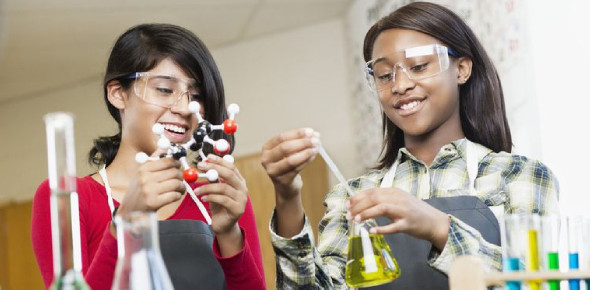30 Questions | Total Attempts: 27133SettingsGrade 9 Science Quiz; Are you a ninth-grader and have a hard time with your science classes? Worry no more, as the quiz below is perfect for helping you revise all that we have covered. Give it a shot and keep an eye out for more tests like this designed to help you pass the final exams. Do share the quiz with your friends.

• 1.
When is an atom stable?
• A.

When it has a full outer orbit.

• B.

When it has the same amount of electrons as protons.

• C.

When it has no neutrons.

• D.

When it has an odd number of valence electrons.

• 2.
An ion is formed when an atom loses/gains an electron.
• A.

True

• B.

False

• 3.
A molecule is the same thing as an atom.
• A.

True

• B.

False

• 4.
How many atoms are in Ca(NO3)2
• A.

Ca-1 N-6 O-2

• B.

Ca-1 N-2 O-6

• C.

Ca-6 N-2 O-1

• D.

Ca-2 N-1 O-6

• 5.
Which does not indicate a chemical change has occurred:
• A.

Change of color

• B.

Heat is produced

• C.

Smell is produced

• D.

Light is produced

• E.

Gas is produced

• F.

Solid is produced

• 6.
What is the equation for photosynthesis?
• A.

H2O+oxygen (light+chlorophyll) = CO2+glucose

• B.

H2O+CO2 (light+chlorophyll) = oxygen+glucose

• C.

CO2+light (chlorophyll +water) = H2O+oxygen

• 7.
Photosynthesis provides us with... (more than one answer)
• A.

Energy

• B.

Water

• C.

Oxygen

• D.

Glucose

• E.

Light

• 8.
Which of the following is not an interaction among ecosystems?
• A.

Competition

• B.

Mutualism

• C.

Equilibrium

• D.

Predation

• 9.
Which of the following is not a limiting factor?
• A.

Climate extremes

• B.

Disease

• C.

Predation

• D.

Biodiversity

• E.

Human disturbances

• F.

Competition

• 10.
_________ is a measure of how many species live in an ecosystem.
• 11.
Bioamplification/biomagnification is when toxin levels get higher at each trophic level.
• A.

True

• B.

False

• 12.
____________ is when toxins build up in a single organism.
• A.

Bioamplification

• B.

Bioaccumulation

• C.

Biomagnification

• 13.
The order of the planets is Mars, Venus, Earth, Mercury, Saturn, Uranus, and Neptune.
• A.

True

• B.

False

• 14.
The Milky Way is a bar-shaped galaxy.
• A.

True

• B.

False

• 15.
A galaxy has more than ___________ stars.
• 16.
A star is made of burning hydrogen and helium.
• A.

True

• B.

False

• 17.
The Moon is not a satellite.
• A.

True

• B.

False

• 18.
A circumpolar constellation can be seen ____________
• A.

Once a year

• B.

All year round

• C.

Never

• D.

Once in every 100 years

• 19.
What are some circumpolar constellations? (more than one)
• A.

Draco

• B.

Aquarius

• C.

Ursa major

• D.

Little dipper

• E.

Leo

• 20.
The longest day of the year is ________
• 21.
Static electricity only happens on conductors.
• A.

True

• B.

False

• 22.
When an atom gains an electron, it becomes:
• A.

Cation

• B.

Anion

• C.

Onion

• 23.
In a circut, the electrons flow from _______ end to ________ end.
• 24.
Why would you get a shock from an object?
• A.

Electrons hate you

• B.

Electrons want to discharge

• C.

• 25.
Which is not a method of discharging?
• A.

At a point

• B.

A spark

• C.

Friction

• D.

Moisture

Related TopicsBack to top